AP Calculus BC Review: Sequences and Convergence

One important topic that shows up on the AP Calculus BC exam (but not on the AB) is sequences. This review article is dedicated to sequences and their convergence properties.

What are Sequences?

Basically, a sequence is just a list of numbers.

However, it’s also much more than that.

We usually study infinite sequences, those that go on forever according to some rule or pattern. Furthermore, we are often interested in determining whether a sequence converges (that is, approaches some fixed value) or not.

Definition and Notation

A sequence is a list of (infinitely many) numbers, called the terms of the sequence.

There are a number of different ways to write a sequence.When n is unspecified, the expression an is called the general term of the sequence.

Moreover, if we know that an = f(n) for some function f, then we say that f(n) is the formula for the general term.

Common Sequences

You have probably seen and worked with many different kinds of sequences already even if you didn’t call them sequences.

1. The natural numbers: 1, 2, 3, 4, 5, …. The formula for the general term is very simple: an = n.
2. The harmonic sequence: 1, 1/2, 1/3, 1/4, 1/5, …. This is nothing more than the sequence of reciprocals of the natural numbers: an = 1/n.
3. The Fibonacci sequence: 1, 1, 2, 3, 5, 8, 13, 21, …. Here, the pattern is to start with two ones, and then to get each new term, we always add the previous two terms together. So 1 + 1 = 2, and then 1 + 2 = 3, and 2 + 3 = 5, and so on.

The Fibonacci sequences is an example of a recursively-defined sequence, because we can write it by the following recursive rule.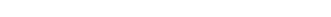By the way, the Fibonacci sequence is important for many reasons, showing up in nature in the most unexpected ways.The nautilus shell grows in the shape of a logarithmic spiral, which is closely related to the Fibonacci sequence.

Convergence and Divergence

We say that a sequence converges to a number a if its terms get arbitrarily close to a the further along in the sequence you get.

To be more precise, we say that the limit (as n → ∞) of the convergent sequence exists (and equals a). Just like limits of functions, we use the “lim” notation.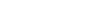Convergence — The Harmonic Sequence

The harmonic sequence (an = 1/n) converges to 0. How can we establish this fact? Well intuitively speaking, if you plug in a very large value of n into the formula 1/n, what do you get? A little experimentation may lead you to the guess that 1/n converges to 0.

If n = 10, you get 1/10 = 0.1, which is already pretty close to 0.

For n = 100, you get 1/100 = 0.01, even closer to 0.

For n = 1,000,000, the value is 1/(1,000,000) = 0.000001, very close to 0 indeed!

The higher n is, the closer 1/n will get to 0. However to rigorously prove this requires a more careful argument.

For our purposes, we will simply state this limit fact without proof.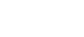.

Divergence — The Natural Number Sequence

If the limit does not exist, then we say that the sequence diverges (or is divergent).

For example, the sequence of natural numbers, 1, 2, 3, 4, 5, …. is divergent because the values simply get larger without bound. There’s no limit to the values, quite literally.

Convergence and Limits

As you can see from the definition, testing the convergence of a sequence requires taking a limit.

There are a few standard tricks to working out these kinds of limits. The key is that the variable n is tending toward infinity (∞), so most of the same techniques that worked to find horizontal asymptotes also work in this new setting.

By the way, now is a great time to review: How do you Find the Horizontal Asymptotes of a Function?

Example

Consider the sequence,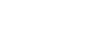.

(a) Write out the first four terms of the sequence.

(b) Determine whether the sequence converges. If it converges, then what is the limit?

Solution

(a) Each term of a sequence can be found by plugging in n-values, similar to how you might find the value of a function f(x) by plugging in x-values.Thus the first four terms are: 3, 2.75, 2, 1.3125.

(b) We need to compute the limit as n approaches infinity.

If you get stuck at this step, imagine how you would handle the problem if it involved x instead of n. For this one, I’d use L’Hospital’s Rule.The last line is based on the fact that the top of the fraction remains constant while the bottom grows without bound as n → ∞.

Furthermore, we can now say that the sequence converges (because the limit does exists), and it converges to 0.

Sequences are Not Series

Now is a great time to remind you of a very important distinction in mathematical terminology.

There is a related concept called a series, which is by definition the sum of a sequence.

In fact, you may already know one important fact. Even though the harmonic sequence converges (to the value 0), the harmonic series actually diverges.

In other words, the sum of all of the reciprocals of natural numbers just grows and grows without bound.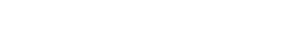For more about the distinction between sequences and series, as well as other topics on the AP Calculus BC exam, check out: AP Calculus BC Cram Sheet

Summary

While sequences make up only a small fraction of the material on an AP Calculus BC exam, they are very important to understand for other topics such as series.

Here are the essential facts you’ll need to know.

1. A sequence is a list of numbers, usually described by a pattern or formula.
2. We call an the general term of the sequence, and if an = f(n), then f is a formula for the general term.
3. A sequence (an) converges to the value a if the following limit statment is true:.
Otherwise we say that the sequence diverges.
4. A series is the sum of a sequence — the two terms are not interchangeable.

Improve your SAT or ACT score, guaranteed. Start your 1 Week Free Trial of Magoosh SAT Prep or your 1 Week Free Trial of Magoosh ACT Prep today!Author

•Shaun earned his Ph. D. in mathematics from The Ohio State University in 2008 (Go Bucks!!). He received his BA in Mathematics with a minor in computer science from Oberlin College in 2002. In addition, Shaun earned a B. Mus. from the Oberlin Conservatory in the same year, with a major in music composition. Shaun still loves music -- almost as much as math! -- and he (thinks he) can play piano, guitar, and bass. Shaun has taught and tutored students in mathematics for about a decade, and hopes his experience can help you to succeed!